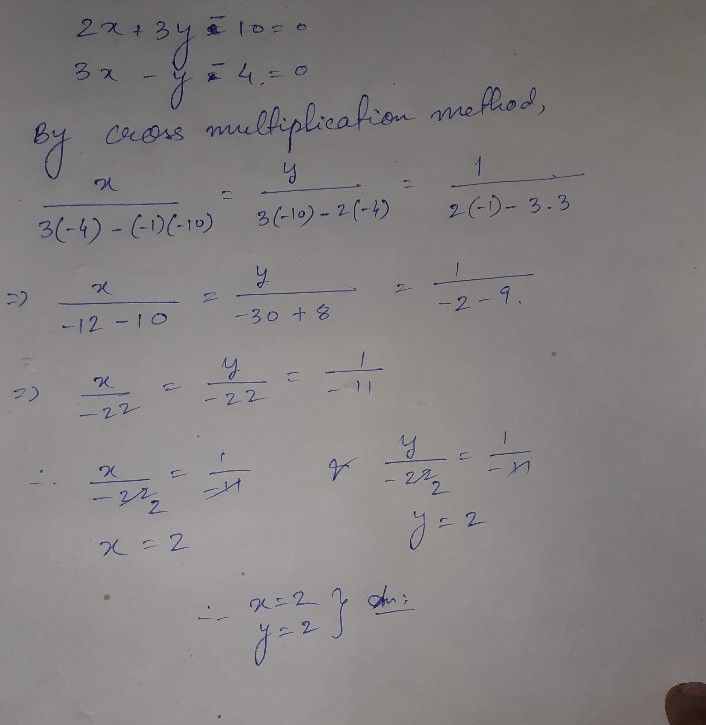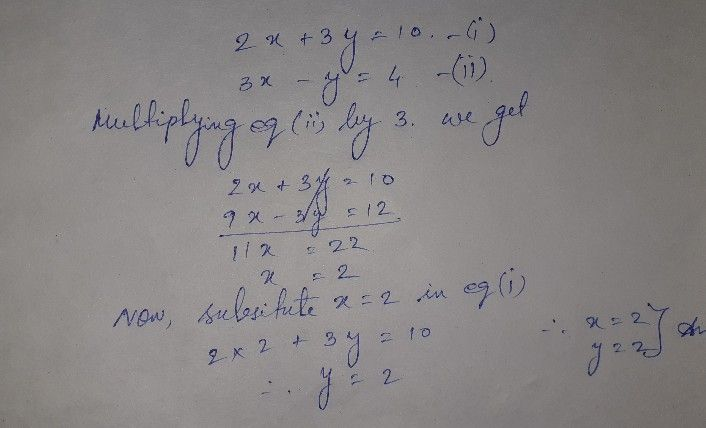Symbol
Problem$CR\square ^{C}$ MULTIPLICATIOiv $2x+3y=10$ $3x$ $-$ $y=$ $21$
Other
SolutionQanda teacher - KAUSHIK DEHope I have helped you.
You can verify my answer my substitution or elimination method
I think you can understand the method of cross multiplication that I have done...generally it looks difficult but its very easy.StudentQanda teacher - KAUSHIK DE
Yes!!Student
fastQanda teacher - KAUSHIK DE
Are you there?Student
yes
the is wrongQanda teacher - KAUSHIK DE
Then what is your doubt ?...tell meStudent
cross multiplication in simple methodQanda teacher - KAUSHIK DE
Its the method of cross multiplication there is no such term in simple method The method of cross multiplication is that which I have doneStudent
okQanda teacher - KAUSHIK DE
Do you want the answer in elimination methodStudent
yesQanda teacher - KAUSHIK DEThis is by elimination method
Hope now it is clear to youStudent
yes
ThnxQanda teacher - KAUSHIK DE
okk..and You are most welcome